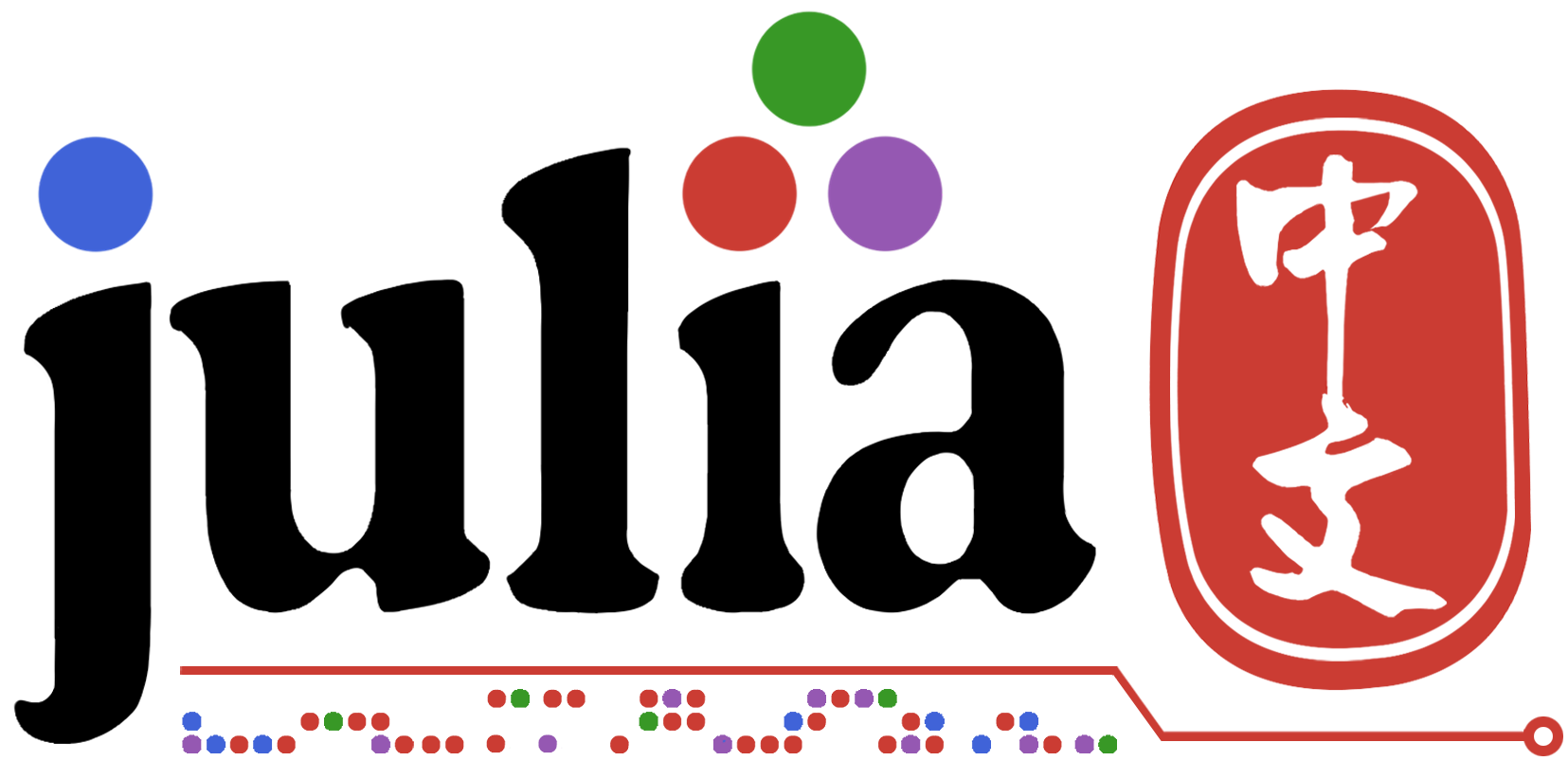# Plots绘图中乱码

``````# code
using Plots
y = rand(20,1)
plot(y,linewidth=2,title="画图")
``````1赞

``````ENV["GKS_ENCODING"] = "utf-8"
``````

## 但是出现了新的问题即保存的图片与显示的图像不同，保存的图像中没有中文，如下所示## 这是第二次用的代码：

``````ENV["GKS_ENCODING"] = "utf-8"
using Plots
y = rand(20,1)
plot(y,linewidth=2,title="画图")
xlabel!("x轴")
ylabel!("y轴")
savefig("plot.png")
``````
1赞

ENV[“GKS_ENCODING”] = “utf-8”